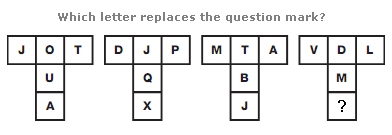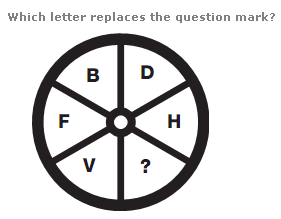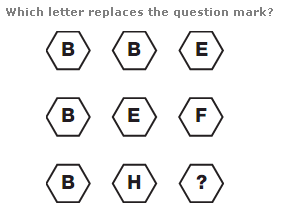# Puzzles - Missing letters puzzles

### Exercise :: Missing letters puzzlesAnswer : V Explanation : Starting with the upper left hand box of the left hand diagram, and moving to the right, letters follow the alphabetical order, 5 letters at a time. A second sequence starts from the middle of this row and goes down, with letters following the alphabetic order, 6 letters at a time. Repeat this pattern for the other diagrams, using steps of 6 and 7 letters, 7 and 8 letters, and 8 and 9 letters.Answer : N Explanation : Starting with B and moving clockwise, letters advance through the alphabet in steps of 2, then 4, 6, 8 and 10.Answer : I Explanation : Convert each letter into its numerical value, and read each row as a 3 digit number. Rows follow the sequence of Square Numbers from 15 to 17.